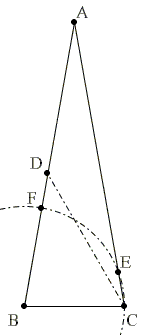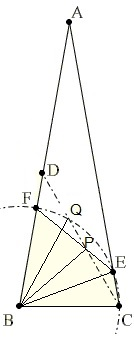# The 80-80-20 Triangle Problem, A Variant, Solution #2

ABC is an isosceles triangle with vertex angle $\angle BAC = 20^{\circ}$ and $AB = AC.$ Draw $\angle BCD = 60^{\circ},$ $D$ lying on $AB.$ Draw an arc with $B$ as center and radius equal to $BC.$ Let this arc cut $AC$ at point $E$ and $AB$ at the point $F.$ Prove that $CE = DF.$### Solution

(This solution is by Hernán Iriarte (Chile).)

$\Delta BEC$ is isosceles with base $\angle BCE=80^\circ.$ It follows that $\angle CBE=20^\circ$ so that $\angle EBF=60^\circ,$ making $\Delta EBF$ equilateral.

Let $CD$ intersect $EF$ at $P$ and the arc at $Q.$$\angle ECP=20^circ=\angle CPE,$ the latter because

$\angle CPE = \angle DFE =\angle BFP-\angle FDP=60^{\circ}-40^{\circ}.$

From here $\angle PBC=40^\circ,$ implying (by SAS) $\Delta BFP=\Delta BCP.$ Further, $\angle EBQ=2\angle ECQ,$ leaving $\angle DBQ=20^{\circ}.$ It then follows that $\Delta FPQ=\Delta CPE$ and, therefore, $FQ=CE.$

Finally, again counting angles, $\Delta FPQ$ is isosceles such that $DF=FQ$ and, by transitivity, also $DF=CE.$